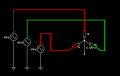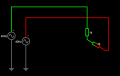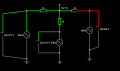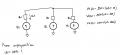# Why there is 0V in 3 phase voltage source in star configuration?

#### Xenon02

Joined Feb 24, 2021
355
Hello !

I've got a question. It might be basic but I want to know.Simulation : https://tinyurl.com/2lhwtdak

Why in the middle it acts like there is 0V ?

Of course when I will remove 1 sourceIt will act like there are 2 source in series with 2 resistor. But I don't know why when there are 3 sources then it will create something like ground.

#### Jony130

Joined Feb 17, 2009
5,405
Try to add L1 + L2 + L3 and you will see that the sum of the instantaneous voltage values in a symmetrical three-phase system is equal to zero.

#### Suncalc

Joined Mar 23, 2021
10
It's the return path for imbalanced currents in the "Y" configuration. Delta circuits are used when driving delta loads, but Y circuits are used when individual loads will be used on each leg. Any imbalance is carried in the return leg. This is why power distribution is done using delta circuits until the last distribution stage where it's converted to Y. This is so single phase transformers can be hung off each leg to supply single pase power at the point of use.

#### LesJones

Joined Jan 8, 2017
3,773
You will only get 0 volts at the star point if the loads on each phase are the same.
If you calculate the instantaneous voltage (With respect to neutral.) at any point on the phase of phase A then if you work out the instantaneous voltages on phase B (+120 degrees) and phase B (+240 degrees.) you will will find the sum of these two voltages equal and opposite to the instantaneous voltage on phase A. As all the loads are the same the star point will be zero volts.

Les.

#### Xenon02

Joined Feb 24, 2021
355
You will only get 0 volts at the star point if the loads on each phase are the same.
If you calculate the instantaneous voltage (With respect to neutral.) at any point on the phase of phase A then if you work out the instantaneous voltages on phase B (+120 degrees) and phase B (+240 degrees.) you will will find the sum of these two voltages equal and opposite to the instantaneous voltage on phase A. As all the loads are the same the star point will be zero volts.

Les.
Superposition ? That's what you meant by instantaneous voltage ?

#### LesJones

Joined Jan 8, 2017
3,773
No The instantaneous voltage at any point on a sine wave is the sine on the angle between the zero crossing point and that point multiplied by the peak voltage of the waveform. The peak voltage of the waveform is root 2 (1.414) times the RMS voltage. (These are star point to phase voltages. NOT phase to phase voltages.)

Les.

#### Xenon02

Joined Feb 24, 2021
355
Try to add L1 + L2 + L3 and you will see that the sum of the instantaneous voltage values in a symmetrical three-phase system is equal to zero.
It doesn't make me confident with this statement. I know only some basics. So I thought maybe it's from them.

No The instantaneous voltage at any point on a sine wave is the sine on the angle between the zero crossing point and that point multiplied by the peak voltage of the waveform. The peak voltage of the waveform is root 2 (1.414) times the RMS voltage. (These are star point to phase voltages. NOT phase to phase voltages.)

Les.
I don't get it.

#### crutschow

Joined Mar 14, 2008
30,463
I don't get it.
Because you don't seem to listening.
Pick any instant in time and sum the voltage from the three phases including their sign at that instant.
Try it for different times.
Tell us what you get.

#### LesJones

Joined Jan 8, 2017
3,773
I was too lazy to draw the three sine waves shifted by 120 degrees so here is a link to a picture I found on the web. I assume that you were taught trigonometry at school so you should know how to calculate the voltage at any point on the waveform. You could create an Exel spreadsheet to display the instantaneous voltage on each phase and the sum of those values at angle increments of say 10 degrees. you will find the sum is always zero.

Les.

#### Xenon02

Joined Feb 24, 2021
355
Because you don't seem to listening.
Pick any instant in time and sum the voltage from the three phases including their sign at that instant.
Try it for different times.
Tell us what you get.
I was too lazy to draw the three sine waves shifted by 120 degrees so here is a link to a picture I found on the web. I assume that you were taught trigonometry at school so you should know how to calculate the voltage at any point on the waveform. You could create an Exel spreadsheet to display the instantaneous voltage on each phase and the sum of those values at angle increments of say 10 degrees. you will find the sum is always zero.

Les.
I mean I get the point where I have to sum L1, L2 and L3.
What did I mean is that when I also sum L1 and L2 and L3 for example here :Okey so L1 + L2 + L3.
224 + 224 - 66 = 127 ?

https://tinyurl.com/2fhy5nvz

#### Jony130

Joined Feb 17, 2009
5,405

#### Xenon02

Joined Feb 24, 2021
355
I was learning in school that if I had for example V1, V2 and V3 with different phases like V1(t) = V1*(wt+0), V2(t) = V2*(wt+120) and V3(t) = (wt+240).
So from this I could make a superposition and calculate the voltage on R1 or R2 or R3. So if R1 was equal V1. The superposition would show it.
I was doing something the same in the school so I thought that maybe there is 0V in that place because R1 = V1, R2 = V2 and R3 = V3. From superposition.As I have mentioned I wasn't very confident with this statement : L1+L2+L3. Because it doesn't work here:Last edited:

#### crutschow

Joined Mar 14, 2008
30,463
Because it doesn't work here:
Because you have a faulty simulation.
If the three sources each have a phase difference of 120 degrees, than how can the two left ones have the same voltage at the same time?
It should be apparent that is not correct.

#### LesJones

Joined Jan 8, 2017
3,773
Example use peak voltage of sinewave = 100 volts

Case 1
Phase 1 is at 0 deg on the waveform. So phase 2 is at 120 deg and phase 3 is at 240 deg.
Instant voltage on phase 1 = 100 x sin 0 = 100 x 0 = 0
Instant voltage on phase 2 = 100 x sin 120 = 100 x 0.866 = 86.6
Instant voltage on phase 3 = 100 x sin 240 = 100 x - 0.866 = -86.6

86.6 + (-86.6) = 0
Now YOU do the calculation for phase 1 at +30 deg.

Les.

•Xenon02

#### WBahn

Joined Mar 31, 2012
26,932
I was learning in school that if I had for example V1, V2 and V3 with different phases like V1(t) = V1*(wt+0), V2(t) = V2*(wt+120) and V3(t) = (wt+240).
So from this I could make a superposition and calculate the voltage on R1 or R2 or R3. So if R1 was equal V1. The superposition would show it.
I was doing something the same in the school so I thought that maybe there is 0V in that place because R1 = V1, R2 = V2 and R3 = V3. From superposition.

View attachment 273419

As I have mentioned I wasn't very confident with this statement : L1+L2+L3. Because it doesn't work here:

View attachment 273420
As others have said, you can't just pick arbitrary values for the three voltage sources and expect the voltage at the star point to be 0V. The conditions that yield this result are when the loads are all the same and the voltage sources have the same amplitude and are 120° apart in phase.

If all else fails, just analyze the circuit. There is nothing magical about a three-phase circuit -- it's just three voltage sources with three loads.

So pick three sources, Va, Vb, and Vc, and then pick three corresponding loads, Ra, Rb, Rc. Declare the voltage at the start point to be Vn and apply KCL to that node. You can then solve for Vn.

Now set all three loads to the same (nonzero) value and you will see that they immediately drop out of the equation with the result being that Vn is now just the sum of the three voltage sources.

Now just set the three voltages to three sinusoidal values that are 120° apart and apply a couple of trig identities and you get that Vn is identically zero at all times.

Last edited:
•Xenon02

#### MrAl

Joined Jun 17, 2014
9,357
Hello !

I've got a question. It might be basic but I want to know.
View attachment 273407

Simulation : https://tinyurl.com/2lhwtdak

Why in the middle it acts like there is 0V ?

Of course when I will remove 1 source View attachment 273408

It will act like there are 2 source in series with 2 resistor. But I don't know why when there are 3 sources then it will create something like ground.
Hello there,

Ground, or zero volts, in electronic circuit theory is an arbitrary choice you can call any of those terminals ground and call it zero volts. If you choose another terminal then the voltage referenced to that terminal will be different than you measure with the choice of ground being the center.

However, there are also industry standards and practices. In industry the center of them is called zero and it make sense because the three currents through the three loads sum to zero, and the choice of ground is usually made on the basis of making other measurements as simple as possible.
So really you should call the center of the three ground as you have been as that makes all the measurements as simple as possible and it's a standard throughout the electrical world, and that node is often referred to as the 'neutral'. I mention this because in many systems the ground wire is not the neutral wire, and would be a separate wire often color coded green.

Now as to the theory of how the voltages sum to zero, it just looks that way because that makes the other three voltages measure the same. The currents in the load however will always sum to zero given a balanced load. If you were to sum the voltages though they would also sum to zero but that's hardly a relevant point except in pure theory.

You do seem to have some questions about this kind of system and if you really want to be able to analyze ANY three phase system, balanced or unbalanced, you can look into describing the AC voltages using complex numbers. If you havent learned that yet then you would have to learn how to deal with complex numbers, but it really isnt difficult if you have a little algebra under your belt already.
The main point here is that if you describe the three line to neutral voltages as complex numbers, the analysis goes just like any other algebraic analysis and it quickly becomes much easier to solve these, and at the same time it is a very general method so you can solve any three phase system. This also reduces your dependence on a bunch of formulas that sooner or later run out when you encounter a formula that doesnt apply to your circuit.
If you want to see this work i can provide an example, but you really need to understand how complex numbers work or you may not get much out of it.

#### WBahn

Joined Mar 31, 2012
26,932
The currents in the load however will always sum to zero given a balanced load.
In his circuit, the currents in the load always sum to zero whether the loads are balanced or not. They have to because his star point is only connected to the loads.

#### LesJones

Joined Jan 8, 2017
3,773
WBahn, I don't agree with your comments in post #19. For the star point to be at the same potential as the neutral (If it exists.) of the supply the loads must be the equal.
I think the TS's lack of understanding of things is that he does not work things out himself to confirm his understanding. He has not responded since I asked him to do the calculation in post #16 for phase 1 at 30 degrees. I would have expected him to do that calculation then try a few more random values.

Les.

Last edited: